# Lecture 8 (1).pptx

29 de May de 2023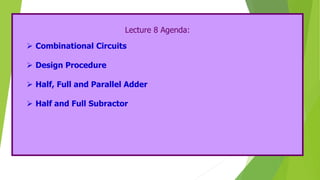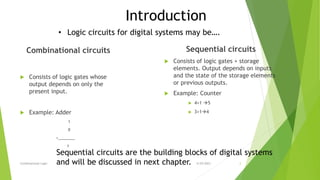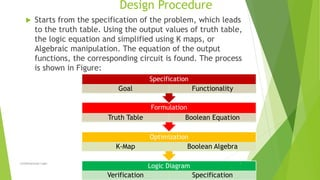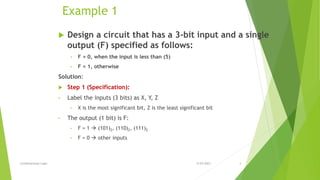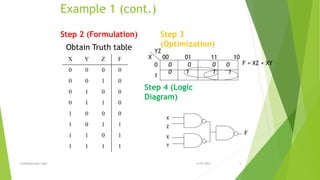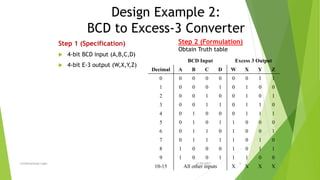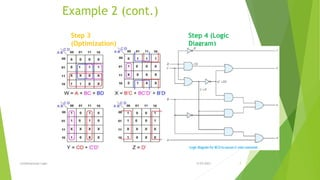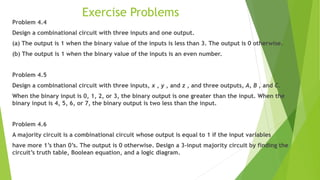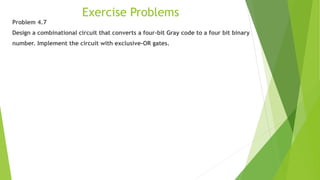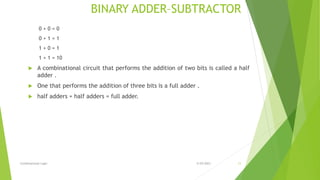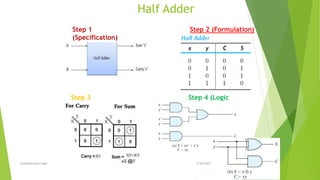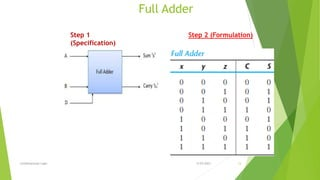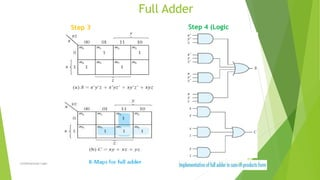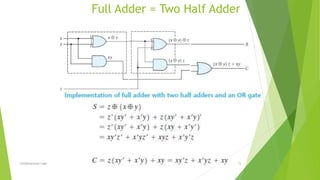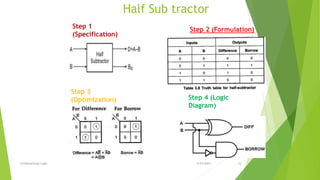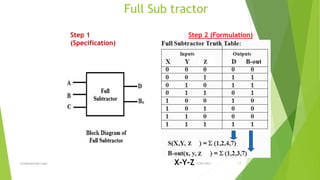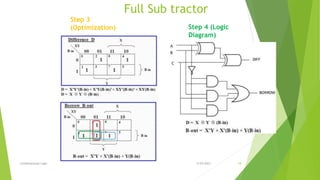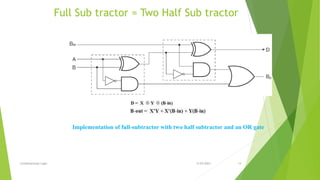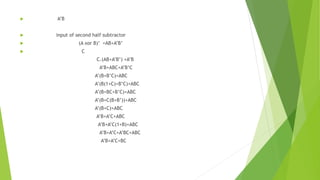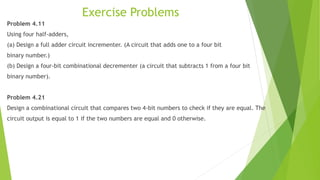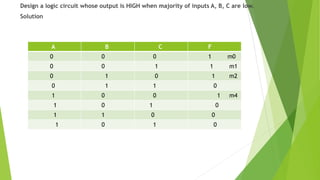1 de 23

### Lecture 8 (1).pptx

• 1. Lecture 8 Agenda:  Combinational Circuits  Design Procedure  Half, Full and Parallel Adder  Half and Full Subractor
• 2. Combinational circuits  Consists of logic gates whose output depends on only the present input.  Example: Adder 1 0 +________ 1 Sequential circuits  Consists of logic gates + storage elements. Output depends on inputs and the state of the storage elements or previous outputs.  Example: Counter  4+1 5  3+14 5/29/2023 Combinational Logic 2 • Logic circuits for digital systems may be…. Introduction Sequential circuits are the building blocks of digital systems and will be discussed in next chapter.
• 3. Design Procedure  Starts from the specification of the problem, which leads to the truth table. Using the output values of truth table, the logic equation and simplified using K maps, or Algebraic manipulation. The equation of the output functions, the corresponding circuit is found. The process is shown in Figure: 5/29/2023 Logic Diagram Verification Specification Optimization K-Map Boolean Algebra Formulation Truth Table Boolean Equation Specification Goal Functionality Combinational Logic 3
• 4. Example 1  Design a circuit that has a 3-bit input and a single output (F) specified as follows: • F = 0, when the input is less than (5) • F = 1, otherwise Solution:  Step 1 (Specification): • Label the inputs (3 bits) as X, Y, Z • X is the most significant bit, Z is the least significant bit • The output (1 bit) is F: • F = 1  (101)2, (110)2, (111)2 • F = 0  other inputs 5/29/2023 Combinational Logic 4
• 5. Step 2 (Formulation) Obtain Truth table X Y Z F 0 0 0 0 0 0 1 0 0 1 0 0 0 1 1 0 1 0 0 0 1 0 1 1 1 1 0 1 1 1 1 1 X YZ 0 1 00 01 11 10 0 0 0 0 0 1 1 1 Step 3 (Optimization) F = XZ + XY X Z X Y F Example 1 (cont.) Step 4 (Logic Diagram) 5/29/2023 Combinational Logic 5
• 6. BCD Input Excess 3 Output Decimal A B C D W X Y Z 0 0 0 0 0 0 0 1 1 1 0 0 0 1 0 1 0 0 2 0 0 1 0 0 1 0 1 3 0 0 1 1 0 1 1 0 4 0 1 0 0 0 1 1 1 5 0 1 0 1 1 0 0 0 6 0 1 1 0 1 0 0 1 7 0 1 1 1 1 0 1 0 8 1 0 0 0 1 0 1 1 9 1 0 0 1 1 1 0 0 10-15 All other inputs X X X X Step 1 (Specification)  4-bit BCD input (A,B,C,D)  4-bit E-3 output (W,X,Y,Z) Design Example 2: BCD to Excess-3 Converter Step 2 (Formulation) Obtain Truth table 5/29/2023 Combinational Logic 6
• 7. Example 2 (cont.) Step 3 (Optimization) Step 4 (Logic Diagram) 5/29/2023 Combinational Logic 7
• 8.  x=B’(C+D)+B (C+D)’   y=CD+(C+D)’  w=A+B(C+D)
• 9. Exercise Problems Problem 4.4 Design a combinational circuit with three inputs and one output. (a) The output is 1 when the binary value of the inputs is less than 3. The output is 0 otherwise. (b) The output is 1 when the binary value of the inputs is an even number. Problem 4.5 Design a combinational circuit with three inputs, x , y , and z , and three outputs, A, B , and C. When the binary input is 0, 1, 2, or 3, the binary output is one greater than the input. When the binary input is 4, 5, 6, or 7, the binary output is two less than the input. Problem 4.6 A majority circuit is a combinational circuit whose output is equal to 1 if the input variables have more 1’s than 0’s. The output is 0 otherwise. Design a 3-input majority circuit by finding the circuit’s truth table, Boolean equation, and a logic diagram.
• 10. Exercise Problems Problem 4.7 Design a combinational circuit that converts a four-bit Gray code to a four bit binary number. Implement the circuit with exclusive-OR gates.
• 11. BINARY ADDER–SUBTRACTOR 0 + 0 = 0 0 + 1 = 1 1 + 0 = 1 1 + 1 = 10  A combinational circuit that performs the addition of two bits is called a half adder .  One that performs the addition of three bits is a full adder .  half adders + half adders = full adder. 5/29/2023 Combinational Logic 11
• 12. Half Adder 5/29/2023 Step 3 (Optimization) Step 4 (Logic Diagram) Step 1 (Specification) Step 2 (Formulation) Combinational Logic 12
• 13. Full Adder 5/29/2023 Step 1 (Specification) Step 2 (Formulation) Combinational Logic 13
• 14. 5/29/2023 Full Adder Step 3 (Optimization) Step 4 (Logic Diagram) Combinational Logic 14
• 15. 5/29/2023 Full Adder = Two Half Adder Combinational Logic 15
• 16. Half Sub tractor 5/29/2023 Step 3 (Optimization) Step 4 (Logic Diagram) Step 1 (Specification) Step 2 (Formulation) Combinational Logic 16
• 17. Full Sub tractor 5/29/2023 Step 1 (Specification) Step 2 (Formulation) X-Y-Z Combinational Logic 17
• 18. 5/29/2023 Full Sub tractor Step 3 (Optimization) Step 4 (Logic Diagram) Combinational Logic 18
• 19. 5/29/2023 Full Sub tractor = Two Half Sub tractor Implementation of full-subtractor with two half subtractor and an OR gate Combinational Logic 19
• 20.  A’B  input of second half subtractor  (A xor B)’ =AB+A’B’  C C.(AB+A’B’) +A’B A’B+ABC+A’B’C A’(B+B’C)+ABC A’(B(1+C)+B’C)+ABC A’(B+BC+B’C)+ABC A’(B+C(B+B’))+ABC A’(B+C)+ABC A’B+A’C+ABC A’B+A’C(1+B)+ABC A’B+A’C+A’BC+ABC A’B+A’C+BC
• 21. Exercise Problems Problem 4.11 Using four half-adders, (a) Design a full adder circuit incrementer. (A circuit that adds one to a four bit binary number.) (b) Design a four-bit combinational decrementer (a circuit that subtracts 1 from a four bit binary number). Problem 4.21 Design a combinational circuit that compares two 4-bit numbers to check if they are equal. The circuit output is equal to 1 if the two numbers are equal and 0 otherwise.
• 22. Design a logic circuit whose output is HIGH when majority of inputs A, B, C are low. Solution A B C F 0 0 0 1 m0 0 0 1 1 m1 0 1 0 1 m2 0 1 1 0 1 0 0 1 m4 1 0 1 0 1 1 0 0 1 0 1 0